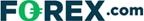# Fibonacci Fans – How Traders Use Them To Construct Support & Resistance Trendlines

Last Updated:

Read on to find out what Fibonacci fans are, how they’re drawn on a chart and what they show traders.

We discuss what different positions of the price-line in relation to the Fibonacci fan trend line show on an example chart.

## What Are Fibonacci Fans?

Fibonacci Fans use Fibonacci ratios based on time and price to construct support and resistance trendlines.

Fibonacci Fans are also used to measure the trend’s movement speed, higher or lower.

### What If The Price Moves Below The Trendline?

If prices move below a Fibonacci Fan trendline, then price is usually expected to fall further until the next Fibonacci Fan trendline level.

Therefore, Fibonacci Fan trendlines are expected to serve as support for up-trending markets (see: Support & Resistance).

### What If The Price Rises To The Fibonacci Fan Line?

Likewise, in a downtrend, if the price rises to a Fibonacci Fan trendline, then that trendline is expected to act as resistance.

If that price is pierced, then the next Fibonacci Fan trendline higher is expected to act as resistance.

The example chart below of the S&P 500 exchange-traded fund (SPY) shows an uptrend that retraced to the 38.2% Fibonacci Fan:

The Fibonacci ratio is also used to predict areas of time in which price might change course.

## Where Can I Use The Fibonacci Fan And Trade Live?

If you are interested in trading using technical analysis, have a look at our reviews of these regulated brokers available in to learn which charting & analysis tools they offer:

CFDs are complex instruments and come with a high risk of losing money rapidly due to leverage. <b>Between 74%-89% of retail investor accounts lose money when trading CFDs.</b> You should consider whether you can afford to take the high risk of losing your money.

Learn more about technical analysis indicators, concepts, and strategies including Momentum, Elliot Waves, Market Thrust, Moving Averages, and more Fibonacci Patterns.

Also see our guides on Forex, Crypto, Stock, CFDs, and Options brokers to find out which tools brokerages offer their clients.

## FAQs

### How do you use a Fibonacci fan in trading?

Traders use a Fibonacci fan (see our example) to determine two things in a chart: support and resistance trendlines, and the trend’s movement speed. Traders do this by drawing a line between two points, the significant support and resistance points within a chosen time frame of the chart.

### What are Fibonacci ratios?

Fibonacci ratios, also known as Fibonacci percentages, are the ratios that show the continuous relationship between the numbers of the Fibonacci sequence. For example, the 1.618 ratio is known as the golden Fibonacci ratio and is used to predict particular patterns in trading, namely support and resistance points.

### Plus500 is not available in the US

Legitimate CFD brokers, like Plus500, cannot accept US clients by law## US traders welcome at these brokers:

### Cryoptocurrencies:• Trade 14+ major crypto coins
• Includes Bitcoin, Ethereum & Ripple
• Super simple setup